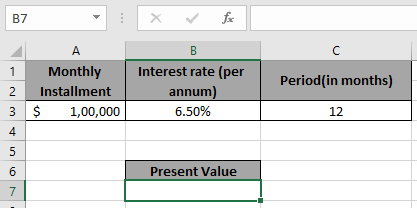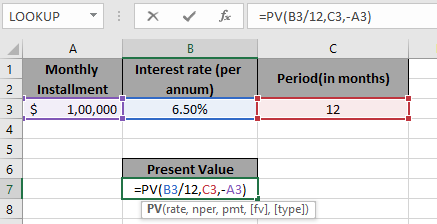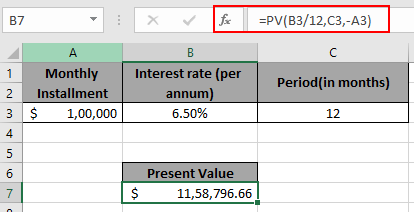# How to Use PV function in Excel

In this article, we will learn about how to find Present Value using PV function in Excel.

PV function returns the present value of the fixed amount paid over a period of time at a constant interest rate.
Syntax:

=PV (rate, nper, pmt, [fv], [type])

rate: Interest rate per period
nper: total no. of payment period.
pmt: amount paid each period.
[fv] - [optional] The present value of future payments must be entered as a negative number.
[type] - [optional] When payments are due. Default is 0.

Use it in example to understand it.

Here we have the amount of \$100,000 is paid every month over a year at a rate of 6.5%.Use the Formula:

=PV( B3/12 , C3 , -A3 )

Explanation:
B3/12: rate is divided by 12 as we are calculating interest for monthly periods.
-A3: amount is in negative so as to get the present value in positive.Press EnterThis means the present value of that amount will be \$1,158,796.66

Hope you understood how to find the present value of the amount using PV formula. Explore more articles here on Accounting. Please feel free to write your queries to us in the comment box below. We will help you.

Related Articles:

How to Use FV function in Excel

How to use XNPV function in Excel

Excel IPMT Function

How to Use Compound Interest Function in Excel

Popular Articles:

How to use the VLOOKUP Function in Excel

How to use the COUNTIF function in Excel 2016

How to Use SUMIF Function in Excel

Terms and Conditions of use

The applications/code on this site are distributed as is and without warranties or liability. In no event shall the owner of the copyrights, or the authors of the applications/code be liable for any loss of profit, any problems or any damage resulting from the use or evaluation of the applications/code.